Mathematical and Physical Journal
for High Schools
Issued by the MATFUND Foundation
 Already signed up? New to KöMaL?

# KöMaL Problems in Physics, March 2014

Show/hide problems of signs:## Problems with sign 'M'

Deadline expired on April 10, 2014.

M. 340. Using the free software called Audacity, determine the coefficient of restitution of a table tennis-ball and multiple different surfaces.

(6 pont)

statistics## Problems with sign 'P'

Deadline expired on April 10, 2014.

P. 4616. Rowers training on Tisza, begin rowing 1.5 hours before the dusk. The speed of the water is 3.6 km/h, and the speed of the rowing boats is 6 m/s with respect to the water. How far from their departure can they row, if they have to arrive back to the dusk?

(3 pont)

solution (in Hungarian), statistics

P. 4617. A student went by bicycle to Saddle City from Frame Village. He planned to go with an average speed of 18 km/h. Because of the heavy traffic, in the first third of his journey his average speed was only 15 km/h.

a) What should his average speed be in the remaining part of his journey, if he is to arrive at the planned time?

b) What should his average speed be in the remaining time, if his speed was 15 km/h in the first one-third of the time of his journey?

(4 pont)

solution (in Hungarian), statistics

P. 4618. In three different containers there are three samples of air at three different temperature values of 300 K, 500 K and 720 K. Allowing heat transfer between the 300 K and the 500 K samples of gas, the common temperature would be 420 K, and allowing heat transfer between the 300 K and the 720 K samples of gas the common temperature would be 600 K. What would be the common temperature of the samples of air of initial temperatures of 500 K and 720 K? What is the ratio of the initial internal energy values of the three samples of gas?

(4 pont)

solution (in Hungarian), statistics

P. 4619. Mary sits on the perimeter of a merry-go-round undergoing uniform circular motion. Matt stands on the ground 12 m from the centre of the merry-go-round. Matt observes Mary coming towards him at a speed of 1 m/s. What is Matt's speed from the point of view of Mary?

(4 pont)

solution (in Hungarian), statistics

P. 4620. A uniform density sphere of radius r=10 cm rolls down with zero initial speed from the top of a hemisphere of radius R=2r. The hemisphere is fixed to a horizontal plane. The coefficient of friction between the two spheres is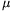=0.2.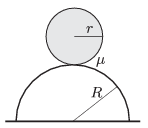a) Where will the rolling sphere begin to slip?

b) What is the speed of the centre of the sphere at the moment when it begins to slip?

(5 pont)

solution (in Hungarian), statistics

P. 4621. A sample of ideal gas is taken through the process shown in the figure. At its initial state V1=2 dm3, p1=105 Pa, T1=300 K. At its final state V2=8 dm3, p2=2.5.104 Pa. What is the highest temperature of the gas during the process and at which state is it reached?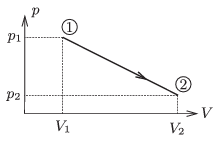(4 pont)

solution (in Hungarian), statistics

P. 4622. N=100 alike galvanic cells are connected to form a battery as shown in the figure. The electromotive force of each cell is U0, and each of them has an internal resistance of Rb. How should the cells be arranged in order that the dissipated power at an external load of resistance R is the greatest, if

aR=Rb;

bR=4Rb;

cR=5Rb?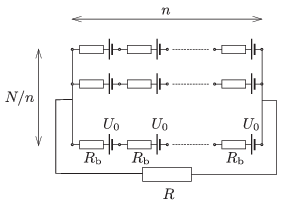(5 pont)

solution (in Hungarian), statistics

P. 4623. A magnetized pin, axed through its centre of mass, swings 24 times in one minute if its axle is vertical. How many swings does the pin make if its axle is horizontal and

a) parallel to the magnetic meridian;

b) perpendicular to the magnetic meridian?

The declination of the magnetic field at the position of the observation is 60o.

(5 pont)

solution (in Hungarian), statistics

P. 4624. Some sparks were observed between the prongs of a fork which was inadvertently put into a microwave oven. The prongs were 5 cm, and the space between them was 4 mm. The frequency of the electromagnetic field was 2.45 GHz. Estimate the magnitude of the electromagnetic induction at the location of the fork. (In the air the maximum electric field that it can tolerate without breakdown is approximately 3 kV/mm.)

(4 pont)

solution (in Hungarian), statistics

P. 4625. The image of the Sun is projected to a white screen by means of a converging lens. What kind of data are necessary, in order to state by what factor the image of the Sun brighter than the part of the screen which is illuminated by the Sun?

(4 pont)

solution (in Hungarian), statistics

P. 4626. There are two alike initially uncharged spheres of radius r at a distance of d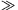r, as shown in the figure.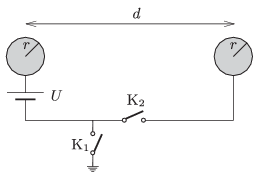a) Switchis closed. What is the force exerted between the two spheres?

b) What would be the force between the two spheres, if in the previous experiment instead of closing switch, switchwas closed?

c) Determine the force exerted between the spheres if both switches are closed.

(6 pont)

solution (in Hungarian), statistics